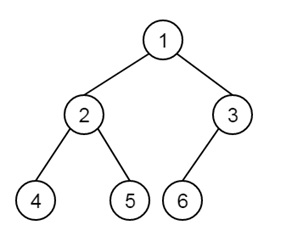# Construct a complete binary tree from given array in level order fashion in C++

Site Under Maintenance - Tutorialspoint# Error. We can’t find the page you’re looking for.

Sorry for the inconvenience. Go to our homepage or check out our Latest Courses, eBooks, Prime Packs...

Back to Home

Suppose we have an array A[], with n elements. We have to construct the binary tree from the array in level order traversal. So the elements from the left in the array will be filled in the tree level-wise starting from level 0. So if the array is like A = [1, 2, 3, 4, 5, 6], then the tree will be like below:If we see closer, we can find that when the parent is present at index i, then its two children will be at index (2i + 1), and (2i + 2). Thus we can insert left and right nodes using its parent nodes. The first element of the array will be the root of the tree.

## Example

Live Demo

#include <iostream>
using namespace std;
class Node {
public:
int data;
Node* left, * right;
};
Node* getNode(int data) {
Node* node = new Node;
node->data = data;
node->left = node->right = NULL;
return node;
}
Node* insertLevelWise(int arr[], Node* root, int i, int n) {
if (i < n) {
Node* temp = getNode(arr[i]);
root = temp;
root->left = insertLevelWise(arr, root->left, 2 * i + 1, n);
root->right = insertLevelWise(arr, root->right, 2 * i + 2, n);
}
return root;
}
void inorderTrav(Node* root) {
if (root != NULL){
inorderTrav(root->left);
cout << root->data <<" ";
inorderTrav(root->right);
}
}
int main() {
int arr[] = { 1, 2, 3, 4, 5, 6};
int n = sizeof(arr)/sizeof(arr);
Node* root = insertLevelWise(arr, root, 0, n);
cout << "Inorder traversal of created tree: ";
inorderTrav(root);
}

## Output

Inorder traversal of created tree: 4 2 5 1 6 3